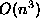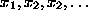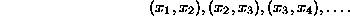Data Structures and Algorithms with Object-Oriented Design Patterns in C## Projects

1.   Design a class that implements the Solution interface defined in Programto represent the nodes of the solution space of a 0/1-knapsack problem described in Section.

Devise a suitable representation for the state of a node and then implement the following properties IsFeasible, IsComplete, Objective, Bound, and Successors. Note, the Successors method returns an IEnumerable object that that represents all the successors of a given node.

1. Use your class with the DepthFirstSolver defined in Programto solve the problem given in Table.
2. Use your class with the BreadthFirstSolver defined in Programto solve the problem given in Table.
3. Use your class with the DepthFirstBranchAndBoundSolver defined in Programto solve the problem given in Table.
2. Do Projectfor the change counting problem described in Section.
3. Do Projectfor the scales balancing problem described in Section.
4. Do Projectfor the N-queens problem described in Exercise.
5. Design and implement a GreedySolver class, along the lines of the DepthFirstSolver and BreadthFirstSolver classes, that conducts a greedy search of the solution space. To do this you will have to add a method to the Solution interface:
```public interface GreedySolution : Solution
{
Solution GreedySuccessor();
}```
6. Design and implement a SimulatedAnnealingSolver class, along the lines of the DepthFirstSolver and BreadthFirstSolver classes, that implements the simulated annealing strategy described in SectionTo do this you will have to add a method to the Solution interface:
```public interface SimulatedAnnealingSolution : Solution
{
Solution RandomSuccessor();
}```
7. Design and implement a dynamic programming algorithm to solve the change counting problem. Your algorithm should always find the optimal solution--even when the greedy algorithm fails.
8. Consider the divide-and-conquer strategy for matrix multiplication described in Section.
1. Rewrite the implementation of the Times method of the Matrix class introduced in Program.
2. Compare the running time of your implementation with thealgorithm given in Program.
9. Consider random number generator that generates random numbers uniformly distributed between zero and one. Such a generator produces a sequence of random numbers. A common test of randomness evaluates the correlation between consecutive pairs of numbers in the sequence. One way to do this is to plot on a graph the points1. Write a program to compute the first 1000 pairs of numbers generated using the UniformRV defined in Program.
2. What conclusions can you draw from your results?Copyright © 2001 by Bruno R. Preiss, P.Eng. All rights reserved.